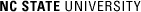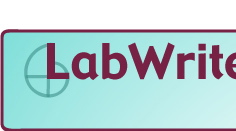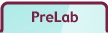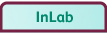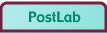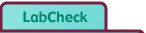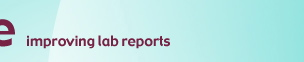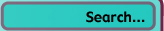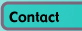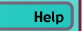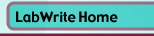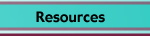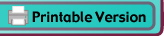# SelfGuide

## PreLab: questions to answer before doing the lab

First, carefully read the description of the lab:

In most lab classes, you will have a lab manual that contains background for the lab and directions for doing the lab procedure. There may also be handouts or other materials you have access to. Read it all. And don't just skim it. In fact, you may need to read it more than once to get a good grasp of it.

### 1. What scientific concept(s) is this lab about?

Identify the scientific concept(s) (principle, theory, law) of the lab and write what you know about the concept(s) from the lab manual, textbook, class notes, handouts, etc.

Most science labs are designed to help you learn about a scientific concept. If you are having trouble identifying the scientific concept this lab is about, check the title of the lab in the lab manual and read the introduction to the lab in the manual. It will be something like photosynthesis, chemical reactions, or inertia. Write down the scientific concept.

Then write down what you know about the concept based on the lab manual, textbook, class notes, and handouts. Don't try to make it pretty; just write it. Get as much down as you can. Because the point of the lab is to learn about the scientific concept, it's important to state what you already know about it.

### 2. What are the objectives for this lab?

Describe the specific actions you are being asked to perform in the lab, such as measure something, analyze something, test something, etc.

Objectives are the activities you are being asked to do in order to complete the lab experiment. Often the objectives are listed in the lab manual. You can list the objectives or write them in a paragraph. If they are not listed in the lab manual, read the lab procedure and figure out from the procedure what the objectives of the lab are. Because objectives are activities, be sure to list them as such: to measure, to analyze, to determine something.

### 3. What is the overall purpose of the lab?

Briefly describe how what you are being asked to do in the lab (the objectives) will help you learn about the lab's scientific concept(s). In other words, show the link between your response to question #2 (what you will do in the lab) to your response to question #1 (what you are supposed to be learning about by doing the lab).

The purpose of the lab is to learn something about the scientific concept the lab is about. This is where you make the all-important link between what you are doing and what you are learning. For example, if the scientific concept is photosynthesis, how will measuring respiration rates help you understand photosynthesis? This is the kind of question you need to ask yourself. Read over the objectives again. They outline what you will be doing in the lab experiment. Describe how you think completing the lab will help you learn about the concept?

### 4. What is your hypothesis for the lab experiment?

First, identify the variables in the experiment. Then state your hypothesis--the relationship or interaction among the variables, the outcome of the experiment you anticipate. Your hypothesis may be stated in 1-2 sentences or sketched out as a graph.

The variables are what you will manipulate (independent variable) and measure (dependent variable) in the lab procedure. The hypothesis is what you anticipate will be the outcome of the procedure, typically the results of the measurements of the dependent variables when the independent variable(s) is manipulated. So the hypothesis is what you expect, based on your understanding of the scientific concept of the lab--what the relationship among the variables will be.

### 5. What reasoning did you use to arrive at your hypothesis?

Explain your hypothesis using the scientific concept of this lab to show the reasoning behind your prediction.

Your hypothesis is a prediction of the outcome of the lab. This prediction is based on your understanding of the scientific concept of the lab. That understanding shapes your prediction of how the lab experiment will turn out, the relationship among variables that you anticipate. Write a paragraph or so describing the logic you used to go from your understanding of the scientific concept to formulating a prediction of the outcomes of the experimental procedure.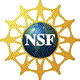© Copyright NC State University 2004 Sponsored and funded by National Science Foundation (DUE-9950405 and DUE-0231086) Site design by Rosa Wallace Rev. MF 5/26/05